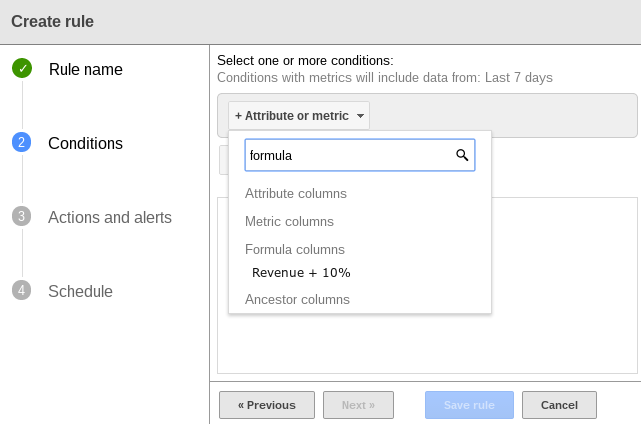# Use formula columns to enhance other Search Ads 360 features

Formula columns can integrate perfectly with other Search Ads 360 features like automated rules, Web Queries, or bid strategies. Check out some of our more advanced use cases below.

Formula Columns + Rules

You can use formula columns as conditions in rules. All of your existing formula columns will be listed alongside the standard Search Ads 360 metrics columns in the condition picker.Use Case

Example

Mix and match date ranges within rules

Create two formula columns:

• One that totals Actions for the past 7 days,

• Another that totals Impressions for the past 30 days.

Then, create a rule that runs if the Actions for the past 7 days are greater than X and Impressions are greater than Y.

Formula column 1
Name: 7-day Actions
Equation:
`Actions.For_date_range(Last_7_days())`

Formula column 2
Name: 30-day Impression
Equation:
`Impressions.For_date_range(Last_30_days())`

Keyword rule
Conditions:
7-day Actions > 300
30-day Impressions > 800
Action:
Change state to Paused

Take action on an object based on average metrics.
Create a formula column that calculates the average clicks per day. Then create a rule that pauses an object or changes a bid if it gets greater than or fewer than X Clicks/Day.

Formula column
Name: Avg. Clicks/Day
Equation:
`Clicks.For_date_range(Last_7_days()) / 7`

Keyword rule
Condition:
Avg. Clicks/Day > 10000
Action:
Change state to Paused

Take action on an object if its metrics are outside a historic range.
Create a formula column that calculates a range of values over a period of time. Then create a rule that pauses an object, changes a bid, or sends a notification if it performs above or below the range.

Formula column
Equation:
`If(Clicks.for_Date_Range(Today()) > (1.15*(Clicks.for_Date_Range(Last_7_days())/7)), "15%+ above Average", Clicks.for_Date_Range(Today()) < (.85*(Clicks.for_Date_Range(Last_7_days())/7)), "<15% below Average", "Within Range")`

Campaign rule
Conditions:
Click Alert equals 15%+ above Average
Click Alert equals <15% below Average
Action:

Simulate “or” values.
Take action on objects with high CPAs, or objects with high costs and zero actions.

Formula column
Name: True CPA
Equation:
`to_money(If(CPA>0, CPA, Cost))`

Keyword rule
Condition:
True CPA > \$50.00
Action:
Pause keyword.

Reference an editable static field -- without editing the rule.
Got a monthly target CPA that changes dramatically? Create a formula column with that metric, and set up rules to alert you if an object misses that target. You can then edit the Formula Column rather than create a whole new rule when the target changes in the next month.

Formula column
Name: Quarterly CPA Goal
Equation:
`CPA = to_money(50)`

Campaign rule
Conditions:
CPA > Quarterly CPA goal
Action:

Formula Columns + Labels

Use Case

Example

Break out related labels into column view for easier sorting and reporting.
Create a formula column that prints a string if an item has specific labels. Then add the column to a report and use it to sort or filter.

Formula column
Name: Brand/Non-Brand
Equation:
`If(Has_label("Brand"), "Brand", Has_label("Generic"), "Generic", Has_label(""), "", "")`

or
Formula column
Name: Search/Remarketing
Equation:
```If(Has_label("Search"), "Search",    Has_label("Remarketing"), "Remarketing", "")```

Formula Columns + Reporting (Budget Pacing, Web Query, etc.)

Use Case

Example

On-the-fly budget pacing.
Estimate what monthly cost might be, factoring in recent fluctuations:

1. Copy the example formula column and update the formula’s
date to the 1st of the most recent month.

3. Change the dates in the Campaign view to  display a recent fluctuation.

Formula column
Name: Projected Monthly Cost From Date Range
Equation:
`to_money(((Cost/num_days())*31) - Cost.For_date_range(Date(2013, 09, 1), Today()))`

Exclude today’s data from “This Month” web queries.
Search Ads 360 updates quickly, but this column excludes today’s data so you’re working only with complete data in your offline reports.

Formula column
Name: Cost - Excluding Today
Equation:
`to_money(Cost - Cost.for_date_range(Today()))`